513.160 Microelectronics and Micromechanics

## Silicon

Silicon is the second most common element in the earth's crust and an important semiconducting material.

### Structural properties

Crystal structure: Diamond
Bravais lattice: face centered cubic
Space group: 227 (F d -3 m),  Strukturbericht: A4,   Pearson symbol: cF8
Point group: m3m (Oh) six 2-fold rotations, four 3-fold rotations, three 4-fold rotations, nine mirror planes, inversion
Lattice constant: $a=0.543$ nm
Atomic weight $28.09$
Atomic density $n_{atoms}=4.995 \times 10^{22}$ 1/cm³
Density $\rho= 2.33$ g/cm³
Density of surface atoms
(100) $6.78 \times 10^{14}$ 1/cm²
(110) $9.59 \times 10^{14}$ 1/cm²
(111) $7.83 \times 10^{14}$ 1/cm²

The primitive lattice vectors are,

$\vec{a}_1=\frac{a}{2}\hat{x}+\frac{a}{2}\hat{y}$,   $\vec{a}_2=\frac{a}{2}\hat{x}+\frac{a}{2}\hat{z}$,   $\vec{a}_3=\frac{a}{2}\hat{y}+\frac{a}{2}\hat{z}$.

There are two atoms in the basis. In fractional coordinates of the conventional unit, the positions of the two atoms are,

$\vec{B}_1=(0,0,0)$,   $\vec{B}_2=(0.25,0.25,0.25)$.

The conventional unit cell is:

$\begin{equation} a = 0.543\, \text{nm}, \, b = 0.543 \,\text{nm}, \, c = 0.543 \,\text{nm}, \, \alpha = 90^{\circ} , \,\beta = 90^{\circ} ,\,\gamma = 90^{\circ} . \end{equation}$

Space group: 227 (F d -3 m)
192 symmetry operations:

1	x,y,z			identity
2	z+1/4,y+1/4,-x+1/4	4-fold screw axis|translation: 0 1/4 0
3	y+1/4,x+1/4,-z+1/4	2-fold screw axis|translation: 1/4 1/4 0
4	x+1/4,z+1/4,-y+1/4	4-fold screw axis|translation: 1/4 0 0
5	z+1/4,x+1/4,-y+1/4	3-bar axis|inversion center at 1/12 1/3 -1/12
6	y+1/4,z+1/4,-x+1/4	3-bar axis|inversion center at 1/3 1/12 -1/12
7	x+1/4,y+1/4,-z+1/4	n-glide plane|translation: 1/4 1/4 0
8	z+1/4,-y+1/4,x+1/4	2-fold screw axis|translation: 1/4 0 1/4
9	y+1/4,-x+1/4,z+1/4	4-fold screw axis|translation: 0 0 1/4
10	x+1/4,-z+1/4,y+1/4	4-fold screw axis|translation: 1/4 0 0
11	z+1/4,-x+1/4,y+1/4	3-bar axis|inversion center at 1/3 -1/12 1/12
12	y+1/4,-z+1/4,x+1/4	3-bar axis|inversion center at 1/12 -1/12 1/3
13	x+1/4,-y+1/4,z+1/4	n-glide plane|translation: 1/4 0 1/4
14	-z+1/4,y+1/4,x+1/4	4-fold screw axis|translation: 0 1/4 0
15	-y+1/4,x+1/4,z+1/4	4-fold screw axis|translation: 0 0 1/4
16	-x+1/4,z+1/4,y+1/4	2-fold screw axis|translation: 0 1/4 1/4
17	-z+1/4,x+1/4,y+1/4	3-bar axis|inversion center at -1/12 1/12 1/3
18	-y+1/4,z+1/4,x+1/4	3-bar axis|inversion center at -1/12 1/3 1/12
19	-x+1/4,y+1/4,z+1/4	n-glide plane|translation: 0 1/4 1/4
20	-z+1/4,-y+1/4,-x+1/4	C2 axis
21	-y+1/4,-x+1/4,-z+1/4	C2 axis
22	-x+1/4,-z+1/4,-y+1/4	C2 axis
23	-z+1/4,-x+1/4,-y+1/4	3-bar axis|inversion center at 1/12 1/12 1/12
24	-y+1/4,-z+1/4,-x+1/4	3-bar axis|inversion center at 1/12 1/12 1/12
25	-x+1/4,-y+1/4,-z+1/4	Ci: 1/12 1/12 1/12
26	-z,-y,x			4-bar axis|inversion center at 0 0 0
27	-y,-x,z			mirror plane
28	-x,-z,y			4-bar axis|inversion center at 0 0 0
29	-z,-x,y			C3 axis
30	-y,-z,x			C3 axis
31	-x,-y,z			C2 axis
32	-z,y,-x			mirror plane
33	-y,x,-z			4-bar axis|inversion center at 0 0 0
34	-x,z,-y			4-bar axis|inversion center at 0 0 0
35	-z,x,-y			C3 axis
36	-y,z,-x			C3 axis
37	-x,y,-z			C2 axis
38	z,-y,-x			4-bar axis|inversion center at 0 0 0
39	y,-x,-z			4-bar axis|inversion center at 0 0 0
40	x,-z,-y			mirror plane
41	z,-x,-y			C3 axis
42	y,-z,-x			C3 axis
43	x,-y,-z			C2 axis
44	z,y,x			mirror plane
45	y,x,z			mirror plane
46	x,z,y			mirror plane
47	z,x,y			C3 axis
48	y,z,x			C3 axis
49	z+1/4,y-1/4,-x-1/4	4-fold screw axis|translation: 0 -1/4 0
50	y+1/4,x-1/4,-z-1/4	C2 axis
51	x+1/4,z-1/4,-y-1/4	4-fold screw axis|translation: 1/4 0 0
52	z+1/4,x-1/4,-y-1/4	3-bar axis|inversion center at 1/12 -1/12 -1/12
53	y+1/4,z-1/4,-x-1/4	3-bar axis|inversion center at -1/12 -1/3 -1/12
54	x+1/4,y-1/4,-z-1/4	n-glide plane|translation: 1/4 -1/4 0
55	z+1/4,-y-1/4,x-1/4	C2 axis
56	y+1/4,-x-1/4,z-1/4	4-fold screw axis|translation: 0 0 -1/4
57	x+1/4,-z-1/4,y-1/4	4-fold screw axis|translation: 1/4 0 0
58	z+1/4,-x-1/4,y-1/4	3-bar axis|inversion center at -1/12 -1/12 -1/3
59	y+1/4,-z-1/4,x-1/4	3-bar axis|inversion center at 1/12 -1/12 -1/12
60	x+1/4,-y-1/4,z-1/4	n-glide plane|translation: 1/4 0 -1/4
61	-z+1/4,y-1/4,x-1/4	4-fold screw axis|translation: 0 -1/4 0
62	-y+1/4,x-1/4,z-1/4	4-fold screw axis|translation: 0 0 -1/4
63	-x+1/4,z-1/4,y-1/4	2-fold screw axis|translation: 0 -1/4 -1/4
64	-z+1/4,x-1/4,y-1/4	3-bar axis|inversion center at 1/3 1/12 -1/12
65	-y+1/4,z-1/4,x-1/4	3-bar axis|inversion center at 1/3 -1/12 1/12
66	-x+1/4,y-1/4,z-1/4	n-glide plane|translation: 0 -1/4 -1/4
67	-z+1/4,-y-1/4,-x-1/4	2-fold screw axis|translation: 1/4 0 -1/4
68	-y+1/4,-x-1/4,-z-1/4	2-fold screw axis|translation: 1/4 -1/4 0
69	-x+1/4,-z-1/4,-y-1/4	C2 axis
70	-z+1/4,-x-1/4,-y-1/4	3-bar axis|inversion center at 1/12 -1/3 1/12
71	-y+1/4,-z-1/4,-x-1/4	3-bar axis|inversion center at 1/12 1/12 -1/3
72	-x+1/4,-y-1/4,-z-1/4	Ci: 1/12 -1/12 -1/12
73	-z,-y+1/2,x+1/2		4-bar axis|inversion center at -1/4 1/4 1/4
74	-y,-x+1/2,z+1/2		g-glide plane|translation: -1/4 1/4 1/2
75	-x,-z+1/2,y+1/2		4-bar axis|inversion center at 0 0 1/2
76	-z,-x+1/2,y+1/2		3-fold screw axis|translation: -1/3 1/3 1/3
77	-y,-z+1/2,x+1/2		C3 axis
78	-x,-y+1/2,z+1/2		2-fold screw axis|translation: 0 0 1/2
79	-z,y+1/2,-x+1/2		g-glide plane|translation: -1/4 1/2 1/4
80	-y,x+1/2,-z+1/2		4-bar axis|inversion center at -1/4 1/4 1/4
81	-x,z+1/2,-y+1/2		4-bar axis|inversion center at 0 1/2 0
82	-z,x+1/2,-y+1/2		C3 axis
83	-y,z+1/2,-x+1/2		3-fold screw axis|translation: -1/3 1/3 1/3
84	-x,y+1/2,-z+1/2		2-fold screw axis|translation: 0 1/2 0
85	z,-y+1/2,-x+1/2		4-bar axis|inversion center at 1/4 1/4 1/4
86	y,-x+1/2,-z+1/2		4-bar axis|inversion center at 1/4 1/4 1/4
87	x,-z+1/2,-y+1/2		mirror plane
88	z,-x+1/2,-y+1/2		C3 axis
89	y,-z+1/2,-x+1/2		C3 axis
90	x,-y+1/2,-z+1/2		C2 axis
91	z,y+1/2,x+1/2		g-glide plane|translation: 1/4 1/2 1/4
92	y,x+1/2,z+1/2		g-glide plane|translation: 1/4 1/4 1/2
93	x,z+1/2,y+1/2		n-glide plane|translation: 0 1/2 1/2
94	z,x+1/2,y+1/2		3-fold screw axis|translation: 1/3 1/3 1/3
95	y,z+1/2,x+1/2		3-fold screw axis|translation: 1/3 1/3 1/3
96	x,y+1/2,z+1/2		translation: 0 1/2 1/2
97	z-1/4,y+1/4,-x-1/4	4-fold screw axis|translation: 0 1/4 0
98	y-1/4,x+1/4,-z-1/4	C2 axis
99	x-1/4,z+1/4,-y-1/4	4-fold screw axis|translation: -1/4 0 0
100	z-1/4,x+1/4,-y-1/4	3-bar axis|inversion center at -1/3 -1/12 -1/12
101	y-1/4,z+1/4,-x-1/4	3-bar axis|inversion center at -1/12 1/12 -1/12
102	x-1/4,y+1/4,-z-1/4	n-glide plane|translation: -1/4 1/4 0
103	z-1/4,-y+1/4,x-1/4	2-fold screw axis|translation: -1/4 0 -1/4
104	y-1/4,-x+1/4,z-1/4	4-fold screw axis|translation: 0 0 -1/4
105	x-1/4,-z+1/4,y-1/4	4-fold screw axis|translation: -1/4 0 0
106	z-1/4,-x+1/4,y-1/4	3-bar axis|inversion center at -1/12 1/3 1/12
107	y-1/4,-z+1/4,x-1/4	3-bar axis|inversion center at 1/12 1/3 -1/12
108	x-1/4,-y+1/4,z-1/4	n-glide plane|translation: -1/4 0 -1/4
109	-z-1/4,y+1/4,x-1/4	4-fold screw axis|translation: 0 1/4 0
110	-y-1/4,x+1/4,z-1/4	4-fold screw axis|translation: 0 0 -1/4
111	-x-1/4,z+1/4,y-1/4	C2 axis
112	-z-1/4,x+1/4,y-1/4	3-bar axis|inversion center at -1/12 1/12 -1/12
113	-y-1/4,z+1/4,x-1/4	3-bar axis|inversion center at -1/12 -1/12 -1/3
114	-x-1/4,y+1/4,z-1/4	n-glide plane|translation: 0 1/4 -1/4
115	-z-1/4,-y+1/4,-x-1/4	C2 axis
116	-y-1/4,-x+1/4,-z-1/4	2-fold screw axis|translation: -1/4 1/4 0
117	-x-1/4,-z+1/4,-y-1/4	2-fold screw axis|translation: 0 1/4 -1/4
118	-z-1/4,-x+1/4,-y-1/4	3-bar axis|inversion center at 1/12 1/12 -1/3
119	-y-1/4,-z+1/4,-x-1/4	3-bar axis|inversion center at -1/3 1/12 1/12
120	-x-1/4,-y+1/4,-z-1/4	Ci: -1/12 1/12 -1/12
121	-z+1/2,-y,x+1/2		4-bar axis|inversion center at 0 0 1/2
122	-y+1/2,-x,z+1/2		g-glide plane|translation: 1/4 -1/4 1/2
123	-x+1/2,-z,y+1/2		4-bar axis|inversion center at 1/4 -1/4 1/4
124	-z+1/2,-x,y+1/2		C3 axis
125	-y+1/2,-z,x+1/2		3-fold screw axis|translation: 1/3 -1/3 1/3
126	-x+1/2,-y,z+1/2		2-fold screw axis|translation: 0 0 1/2
127	-z+1/2,y,-x+1/2		mirror plane
128	-y+1/2,x,-z+1/2		4-bar axis|inversion center at 1/4 1/4 1/4
129	-x+1/2,z,-y+1/2		4-bar axis|inversion center at 1/4 1/4 1/4
130	-z+1/2,x,-y+1/2		C3 axis
131	-y+1/2,z,-x+1/2		C3 axis
132	-x+1/2,y,-z+1/2		C2 axis
133	z+1/2,-y,-x+1/2		4-bar axis|inversion center at 1/2 0 0
134	y+1/2,-x,-z+1/2		4-bar axis|inversion center at 1/4 -1/4 1/4
135	x+1/2,-z,-y+1/2		g-glide plane|translation: 1/2 -1/4 1/4
136	z+1/2,-x,-y+1/2		3-fold screw axis|translation: 1/3 -1/3 1/3
137	y+1/2,-z,-x+1/2		C3 axis
138	x+1/2,-y,-z+1/2		2-fold screw axis|translation: 1/2 0 0
139	z+1/2,y,x+1/2		n-glide plane|translation: 1/2 0 1/2
140	y+1/2,x,z+1/2		g-glide plane|translation: 1/4 1/4 1/2
141	x+1/2,z,y+1/2		g-glide plane|translation: 1/2 1/4 1/4
142	z+1/2,x,y+1/2		3-fold screw axis|translation: 1/3 1/3 1/3
143	y+1/2,z,x+1/2		3-fold screw axis|translation: 1/3 1/3 1/3
144	x+1/2,y,z+1/2		translation: 1/2 0 1/2
145	z-1/4,y-1/4,-x+1/4	4-fold screw axis|translation: 0 -1/4 0
146	y-1/4,x-1/4,-z+1/4	2-fold screw axis|translation: -1/4 -1/4 0
147	x-1/4,z-1/4,-y+1/4	4-fold screw axis|translation: -1/4 0 0
148	z-1/4,x-1/4,-y+1/4	3-bar axis|inversion center at 1/12 -1/12 1/3
149	y-1/4,z-1/4,-x+1/4	3-bar axis|inversion center at -1/12 1/12 1/3
150	x-1/4,y-1/4,-z+1/4	n-glide plane|translation: -1/4 -1/4 0
151	z-1/4,-y-1/4,x+1/4	C2 axis
152	y-1/4,-x-1/4,z+1/4	4-fold screw axis|translation: 0 0 1/4
153	x-1/4,-z-1/4,y+1/4	4-fold screw axis|translation: -1/4 0 0
154	z-1/4,-x-1/4,y+1/4	3-bar axis|inversion center at -1/12 -1/12 1/12
155	y-1/4,-z-1/4,x+1/4	3-bar axis|inversion center at -1/3 -1/12 -1/12
156	x-1/4,-y-1/4,z+1/4	n-glide plane|translation: -1/4 0 1/4
157	-z-1/4,y-1/4,x+1/4	4-fold screw axis|translation: 0 -1/4 0
158	-y-1/4,x-1/4,z+1/4	4-fold screw axis|translation: 0 0 1/4
159	-x-1/4,z-1/4,y+1/4	C2 axis
160	-z-1/4,x-1/4,y+1/4	3-bar axis|inversion center at -1/12 -1/3 -1/12
161	-y-1/4,z-1/4,x+1/4	3-bar axis|inversion center at -1/12 -1/12 1/12
162	-x-1/4,y-1/4,z+1/4	n-glide plane|translation: 0 -1/4 1/4
163	-z-1/4,-y-1/4,-x+1/4	2-fold screw axis|translation: -1/4 0 1/4
164	-y-1/4,-x-1/4,-z+1/4	C2 axis
165	-x-1/4,-z-1/4,-y+1/4	2-fold screw axis|translation: 0 -1/4 1/4
166	-z-1/4,-x-1/4,-y+1/4	3-bar axis|inversion center at -1/3 1/12 1/12
167	-y-1/4,-z-1/4,-x+1/4	3-bar axis|inversion center at 1/12 -1/3 1/12
168	-x-1/4,-y-1/4,-z+1/4	Ci: -1/12 -1/12 1/12
169	-z+1/2,-y+1/2,x		4-bar axis|inversion center at 1/4 1/4 1/4
170	-y+1/2,-x+1/2,z		mirror plane
171	-x+1/2,-z+1/2,y		4-bar axis|inversion center at 1/4 1/4 1/4
172	-z+1/2,-x+1/2,y		C3 axis
173	-y+1/2,-z+1/2,x		C3 axis
174	-x+1/2,-y+1/2,z		C2 axis
175	-z+1/2,y+1/2,-x		g-glide plane|translation: 1/4 1/2 -1/4
176	-y+1/2,x+1/2,-z		4-bar axis|inversion center at 0 1/2 0
177	-x+1/2,z+1/2,-y		4-bar axis|inversion center at 1/4 1/4 -1/4
178	-z+1/2,x+1/2,-y		3-fold screw axis|translation: 1/3 1/3 -1/3
179	-y+1/2,z+1/2,-x		C3 axis
180	-x+1/2,y+1/2,-z		2-fold screw axis|translation: 0 1/2 0
181	z+1/2,-y+1/2,-x		4-bar axis|inversion center at 1/4 1/4 -1/4
182	y+1/2,-x+1/2,-z		4-bar axis|inversion center at 1/2 0 0
183	x+1/2,-z+1/2,-y		g-glide plane|translation: 1/2 1/4 -1/4
184	z+1/2,-x+1/2,-y		C3 axis
185	y+1/2,-z+1/2,-x		3-fold screw axis|translation: 1/3 1/3 -1/3
186	x+1/2,-y+1/2,-z		2-fold screw axis|translation: 1/2 0 0
187	z+1/2,y+1/2,x		g-glide plane|translation: 1/4 1/2 1/4
188	y+1/2,x+1/2,z		n-glide plane|translation: 1/2 1/2 0
189	x+1/2,z+1/2,y		g-glide plane|translation: 1/2 1/4 1/4
190	z+1/2,x+1/2,y		3-fold screw axis|translation: 1/3 1/3 1/3
191	y+1/2,z+1/2,x		3-fold screw axis|translation: 1/3 1/3 1/3
192	x+1/2,y+1/2,z		translation: 1/2 1/2 0


### Phonons

The phonon dispersion relation for silicon is shown below. There are two atoms in the basis so there are three acoustic branches and three optical branches. The phonon density of states is plotted in the right panel.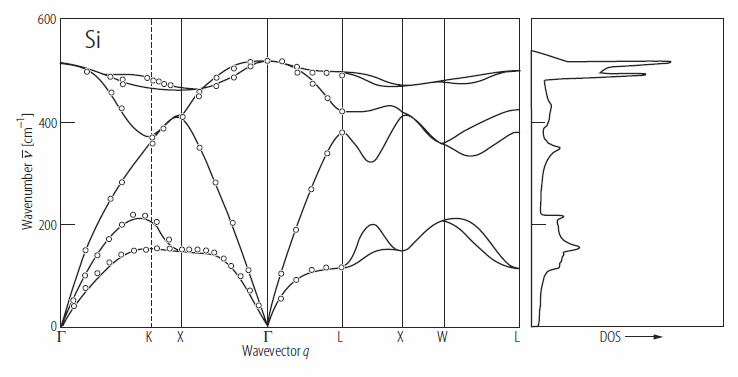Source: O. Madelung, U. R�ssler, M. Schulz (ed.) SpringerMaterials, Silicon (Si) phonon dispersion relations Landolt-B�rnstein - Group III Condensed Matter 41A1a (Group IV Elements, IV-IV and III-V Compounds. Part a - Lattice Properties) http://materials.springer.com/lb/docs/sm_lbs_978-3-540-31355-7_200 10.1007/10551045_200 (Springer-Verlag Berlin Heidelberg �2001) Accessed: 14-01-2016.The density of phonon modes is three times the density of atoms. Integrating over the phonon density of states should be the number of degrees of freedom in a cubic meter of Si. The integral of the data above is 1.498 × 1029. The density of silicon is 2.33 g/cm³ = 2330 kg/m³. The mass of a silicon atom is 4.664 × 10-26 kg. The number of silicon atoms in a cubic meter is 4.99 × 1028. Multipying this by 3 to get the number of degrees of freedom yields 1.498 × 1029 so this density of states is consistent with the known number of vibrational modes in silicon.

The energy spectral density specifies which phonon modes are occupied for a specific temperature. This is analogous to the Planck radiation curves for blackbody radiation. The energy spectral density is plotted for temperatures $T=60i\quad\text{[K]}\quad i=1,2,\cdots,10$.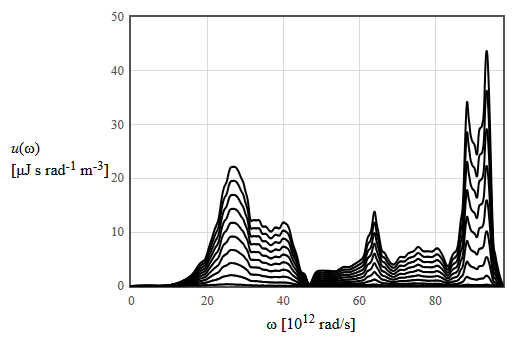The integral of the energy spectral density over all frequencies is the phonon contribution to the internal energy density.The derivative of the internal energy with respect to temperature is the phonon contribution to the specific heat, $c_v=\frac{du}{dT}$.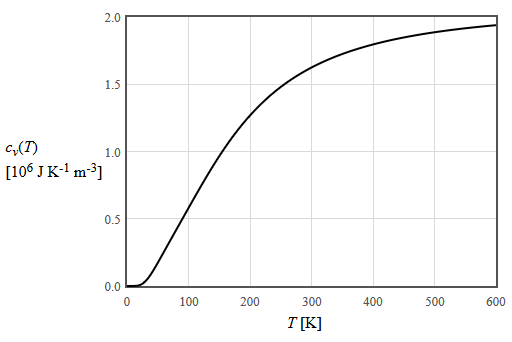The phonon contribution to the entropy density can be calculated from the specific heat $s=\int\frac{c_v}{T}dT$.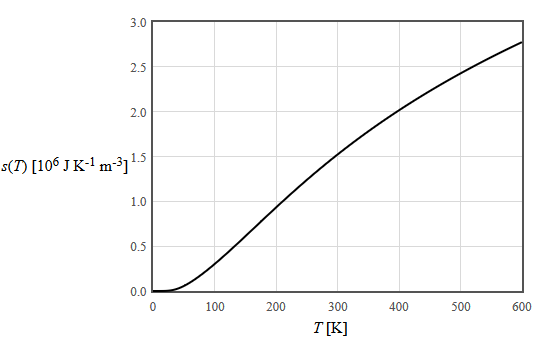A crystal held at constant temperature will go to a minimum of the Helmholtz free energy, $f=u-Ts$.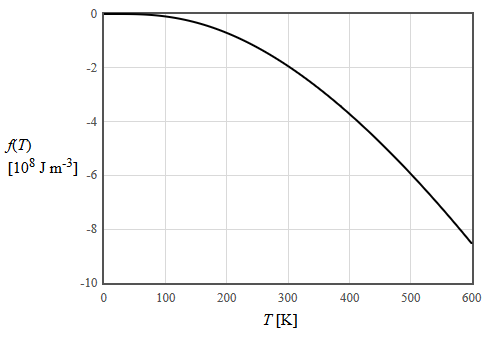Data for the Gibbs free energy, $G=U+pV-TS$, for silicon is available from the SGTE pure element database. The Gibbs energies of different phases of silicon are plotted below.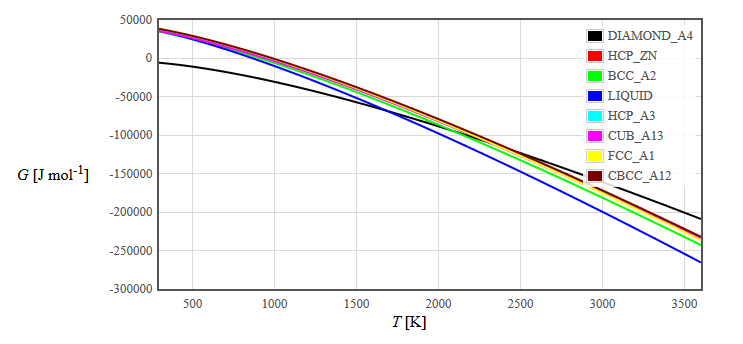$\begin{equation} G_{\text{diamond A4}}(T) = \begin{cases} -8162.609+137.236859T-22.8317533T\ln(T)-1.912904\times 10^{-3}T^2-0.003552\times 10^{-6}T^3+176667/T, & \mbox{for } 298.15 \lt T \lt 1687 \text{ [K]} \\ -9457.642+167.281367T-27.196T\ln(T)-420.369\times 10^{28}/T^{9}. &\mbox{for } 1687 \lt T \lt 3600 \text{ [K]} \end{cases} \end{equation}$ $\begin{equation} G_{\text{LIQUID}}(T) = \begin{cases} 42533.751+107.13742T-22.8317533T\ln(T)-1.912904\times 10^{-3}T^2-0.003552\times 10^{-6}T^3+176667/T+209.307\times 10^{-23}T^7, & \mbox{for } 298.15 \lt T \lt 1687 \text{ [K]} \\ 40370.523+137.722298T-27.196T\ln(T). &\mbox{for } 1687 \lt T \lt 3600 \text{ [K]} \end{cases} \end{equation}$

### Electrical Band Structure

Silicon is an indirect bandgap semiconductor.

The six 6 conduction band minima can be approximated by the paraboloids,

$\begin{equation} E_{c100}=E_g+\frac{\hbar^2}{2m_l}\left(k_x-\frac{1.7\pi}{a}\right)^2+\frac{\hbar^2}{2m_t}k_y^2+\frac{\hbar^2}{2m_t}k_z^2, \end{equation}$ $\begin{equation} E_{c\overline{1}00}=E_g+\frac{\hbar^2}{2m_l}\left(k_x+\frac{1.7\pi}{a}\right)^2+\frac{\hbar^2}{2m_t}k_y^2+\frac{\hbar^2}{2m_t}k_z^2, \end{equation}$ $\begin{equation} E_{c010}=E_g+\frac{\hbar^2}{2m_t}k_x^2+\frac{\hbar^2}{2m_l}\left(k_y-\frac{1.7\pi}{a}\right)^2+\frac{\hbar^2}{2m_t}k_z^2, \end{equation}$ $\begin{equation} E_{c0\overline{1}0}=E_g+\frac{\hbar^2}{2m_t}k_x^2+\frac{\hbar^2}{2m_l}\left(k_y+\frac{1.7\pi}{a}\right)^2+\frac{\hbar^2}{2m_t}k_z^2, \end{equation}$ $\begin{equation} E_{c001}=E_g+\frac{\hbar^2}{2m_t}k_x^2+\frac{\hbar^2}{2m_t}k_y^2+\frac{\hbar^2}{2m_l}\left(k_z-\frac{1.7\pi}{a}\right)^2, \end{equation}$ $\begin{equation} E_{c00\overline{1}}=E_g+\frac{\hbar^2}{2m_t}k_x^2+\frac{\hbar^2}{2m_t}k_y^2+\frac{\hbar^2}{2m_l}\left(k_z+\frac{1.7\pi}{a}\right)^2. \end{equation}$

Here $a = 0.543$ nm is the lattice constant, $m_t = 0.19m_e$ is the transverse electron effective mass, $m_l = 0.98m_e$ is the longitudinal electron effective mass, and $m_e = 9.11\times 10^{-31}$ kg is the mass of an electron.

The valence bands consist of a light hole band, a heavy hole band, and a split-off band. All three bands have a maximum at $k=0$. The light hole band and the heavy hole band are degenerate at $k=0$ while the energy of the split-off band is $E_{so}$ lower at $k=0$. The dispersion for the light holes and the heavy holes are given approximately by, [Askerov]

$\begin{equation} E_{v,lh}=-\frac{\hbar^2}{2m_{e}}\left(4.1k^2-\sqrt{1.21k^4+4.1(k_x^2k_y^2+k_x^2k_z^2+k_y^2k_z^2)}\right), \end{equation}$

and

$\begin{equation} E_{v,hh}=-\frac{\hbar^2}{2m_{e}}\left(4.1k^2+\sqrt{1.21k^4+4.1(k_x^2k_y^2+k_x^2k_z^2+k_y^2k_z^2)}\right), \end{equation}$

These bands are quadratic but anisotropic. They are sometimes approximated by isotropic bands described by effective masses,

$\begin{equation} E_{v,lh}\approx -\frac{\hbar^2k^2}{2m_{lh}}, \end{equation}$

and

$\begin{equation} E_{v,hh}\approx -\frac{\hbar^2k^2}{2m_{hh}}. \end{equation}$

The split-off band is isotropic,

$\begin{equation} E_{v,so}=-E_{so}-\frac{\hbar^2k^2}{2m_{so}}. \end{equation}$

For silicon, $m_{lh}= 0.16m_e$, $m_{hh}= 0.49m_e$, $m_{so}= 0.24m_e$, and $E_{so} = 0.035\,\text{eV}$.

The electron density of states for silicon is shown below. At temperature $T=0$ the valence band is occupied and the conduction band is empty. At room temperature, a few electrons are thermally excited into the conduction band and they leave and equal number of holes behind in the valence band.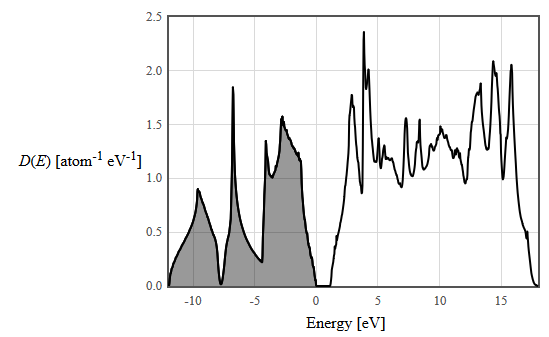Near the top of the valence band, the density of states have the form: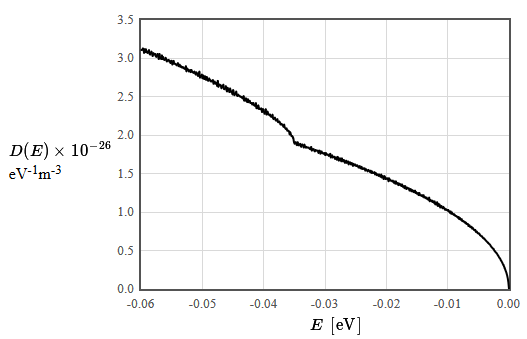$E$ [eV]  $D(E)$ [eV-1m-3] -0.06 3.10E+26 -0.059899833 3.11E+26 -0.059799666 3.11E+26 -0.059699499 3.13E+26 -0.059599332 3.12E+26 -0.059499165 3.07E+26 -0.059398998 3.12E+26 -0.059298831 3.08E+26 -0.059198664 3.12E+26 -0.059098497 3.08E+26 -0.058998331 3.09E+26 -0.058898164 3.07E+26 -0.058797997 3.08E+26 -0.05869783 3.11E+26 -0.058597663 3.06E+26 -0.058497496 3.05E+26 -0.058397329 3.07E+26 -0.058297162 3.09E+26 -0.058196995 3.06E+26 -0.058096828 3.03E+26 -0.057996661 3.07E+26 -0.057896494 3.06E+26 -0.057796327 3.05E+26 -0.05769616 3.06E+26 -0.057595993 3.03E+26 -0.057495826 3.02E+26 -0.057395659 3.04E+26 -0.057295492 3.06E+26 -0.057195326 3.02E+26 -0.057095159 2.99E+26 -0.056994992 3.06E+26 -0.056894825 3.01E+26 -0.056794658 3.01E+26 -0.056694491 2.99E+26 -0.056594324 3.04E+26 -0.056494157 2.99E+26 -0.05639399 3.02E+26 -0.056293823 2.99E+26 -0.056193656 2.98E+26 -0.056093489 2.98E+26 -0.055993322 3.01E+26 -0.055893155 2.99E+26 -0.055792988 2.96E+26 -0.055692821 2.97E+26 -0.055592654 2.98E+26 -0.055492487 2.98E+26 -0.055392321 2.96E+26 -0.055292154 2.97E+26 -0.055191987 2.94E+26 -0.05509182 2.96E+26 -0.054991653 2.97E+26 -0.054891486 2.92E+26 -0.054791319 2.93E+26 -0.054691152 2.97E+26 -0.054590985 2.93E+26 -0.054490818 2.93E+26 -0.054390651 2.93E+26 -0.054290484 2.92E+26 -0.054190317 2.91E+26 -0.05409015 2.92E+26 -0.053989983 2.94E+26 -0.053889816 2.90E+26 -0.053789649 2.90E+26 -0.053689482 2.92E+26 -0.053589316 2.89E+26 -0.053489149 2.90E+26 -0.053388982 2.89E+26 -0.053288815 2.88E+26 -0.053188648 2.89E+26 -0.053088481 2.88E+26 -0.052988314 2.87E+26 -0.052888147 2.87E+26 -0.05278798 2.86E+26 -0.052687813 2.90E+26 -0.052587646 2.83E+26 -0.052487479 2.87E+26 -0.052387312 2.86E+26 -0.052287145 2.85E+26 -0.052186978 2.83E+26 -0.052086811 2.84E+26 -0.051986644 2.85E+26 -0.051886477 2.82E+26 -0.051786311 2.85E+26 -0.051686144 2.83E+26 -0.051585977 2.82E+26 -0.05148581 2.82E+26 -0.051385643 2.81E+26 -0.051285476 2.83E+26 -0.051185309 2.81E+26 -0.051085142 2.79E+26 -0.050984975 2.82E+26 -0.050884808 2.81E+26 -0.050784641 2.78E+26 -0.050684474 2.77E+26 -0.050584307 2.80E+26 -0.05048414 2.83E+26 -0.050383973 2.75E+26 -0.050283806 2.77E+26 -0.050183639 2.77E+26 -0.050083472 2.79E+26 -0.049983306 2.74E+26 -0.049883139 2.78E+26 -0.049782972 2.74E+26 -0.049682805 2.73E+26 -0.049582638 2.77E+26 -0.049482471 2.74E+26 -0.049382304 2.72E+26 -0.049282137 2.76E+26 -0.04918197 2.74E+26 -0.049081803 2.71E+26 -0.048981636 2.71E+26 -0.048881469 2.74E+26 -0.048781302 2.72E+26 -0.048681135 2.71E+26 -0.048580968 2.70E+26 -0.048480801 2.71E+26 -0.048380634 2.70E+26 -0.048280467 2.72E+26 -0.048180301 2.67E+26 -0.048080134 2.68E+26 -0.047979967 2.70E+26 -0.0478798 2.67E+26 -0.047779633 2.68E+26 -0.047679466 2.68E+26 -0.047579299 2.64E+26 -0.047479132 2.71E+26 -0.047378965 2.63E+26 -0.047278798 2.66E+26 -0.047178631 2.66E+26 -0.047078464 2.65E+26 -0.046978297 2.65E+26 -0.04687813 2.61E+26 -0.046777963 2.64E+26 -0.046677796 2.63E+26 -0.046577629 2.66E+26 -0.046477462 2.60E+26 -0.046377295 2.60E+26 -0.046277129 2.61E+26 -0.046176962 2.64E+26 -0.046076795 2.59E+26 -0.045976628 2.57E+26 -0.045876461 2.62E+26 -0.045776294 2.59E+26 -0.045676127 2.59E+26 -0.04557596 2.56E+26 -0.045475793 2.61E+26 -0.045375626 2.58E+26 -0.045275459 2.56E+26 -0.045175292 2.57E+26 -0.045075125 2.53E+26 -0.044974958 2.59E+26 -0.044874791 2.56E+26 -0.044774624 2.52E+26 -0.044674457 2.54E+26 -0.04457429 2.54E+26 -0.044474124 2.57E+26 -0.044373957 2.52E+26 -0.04427379 2.51E+26 -0.044173623 2.53E+26 -0.044073456 2.52E+26 -0.043973289 2.51E+26 -0.043873122 2.51E+26 -0.043772955 2.50E+26 -0.043672788 2.50E+26 -0.043572621 2.50E+26 -0.043472454 2.48E+26 -0.043372287 2.48E+26 -0.04327212 2.51E+26 -0.043171953 2.45E+26 -0.043071786 2.48E+26 -0.042971619 2.45E+26 -0.042871452 2.48E+26 -0.042771285 2.45E+26 -0.042671119 2.43E+26 -0.042570952 2.47E+26 -0.042470785 2.43E+26 -0.042370618 2.44E+26 -0.042270451 2.45E+26 -0.042170284 2.42E+26 -0.042070117 2.42E+26 -0.04196995 2.42E+26 -0.041869783 2.40E+26 -0.041769616 2.42E+26 -0.041669449 2.42E+26 -0.041569282 2.39E+26 -0.041469115 2.39E+26 -0.041368948 2.38E+26 -0.041268781 2.42E+26 -0.041168614 2.35E+26 -0.041068447 2.38E+26 -0.04096828 2.35E+26 -0.040868114 2.39E+26 -0.040767947 2.34E+26 -0.04066778 2.35E+26 -0.040567613 2.34E+26 -0.040467446 2.34E+26 -0.040367279 2.35E+26 -0.040267112 2.30E+26 -0.040166945 2.34E+26 -0.040066778 2.34E+26 -0.039966611 2.31E+26 -0.039866444 2.28E+26 -0.039766277 2.30E+26 -0.03966611 2.34E+26 -0.039565943 2.27E+26 -0.039465776 2.27E+26 -0.039365609 2.31E+26 -0.039265442 2.28E+26 -0.039165275 2.27E+26 -0.039065109 2.26E+26 -0.038964942 2.27E+26 -0.038864775 2.25E+26 -0.038764608 2.24E+26 -0.038664441 2.24E+26 -0.038564274 2.27E+26 -0.038464107 2.22E+26 -0.03836394 2.21E+26 -0.038263773 2.22E+26 -0.038163606 2.23E+26 -0.038063439 2.20E+26 -0.037963272 2.19E+26 -0.037863105 2.20E+26 -0.037762938 2.21E+26 -0.037662771 2.17E+26 -0.037562604 2.17E+26 -0.037462437 2.18E+26 -0.03736227 2.17E+26 -0.037262104 2.14E+26 -0.037161937 2.15E+26 -0.03706177 2.14E+26 -0.036961603 2.14E+26 -0.036861436 2.13E+26 -0.036761269 2.11E+26 -0.036661102 2.14E+26 -0.036560935 2.10E+26 -0.036460768 2.09E+26 -0.036360601 2.08E+26 -0.036260434 2.09E+26 -0.036160267 2.06E+26 -0.0360601 2.06E+26 -0.035959933 2.06E+26 -0.035859766 2.05E+26 -0.035759599 2.01E+26 -0.035659432 2.04E+26 -0.035559265 2.01E+26 -0.035459098 1.99E+26 -0.035358932 1.99E+26 -0.035258765 1.98E+26 -0.035158598 1.96E+26 -0.035058431 1.91E+26 -0.034958264 1.89E+26 -0.034858097 1.91E+26 -0.03475793 1.91E+26 -0.034657763 1.88E+26 -0.034557596 1.87E+26 -0.034457429 1.91E+26 -0.034357262 1.87E+26 -0.034257095 1.87E+26 -0.034156928 1.88E+26 -0.034056761 1.89E+26 -0.033956594 1.86E+26 -0.033856427 1.85E+26 -0.03375626 1.88E+26 -0.033656093 1.88E+26 -0.033555927 1.86E+26 -0.03345576 1.84E+26 -0.033355593 1.88E+26 -0.033255426 1.85E+26 -0.033155259 1.84E+26 -0.033055092 1.85E+26 -0.032954925 1.84E+26 -0.032854758 1.84E+26 -0.032754591 1.84E+26 -0.032654424 1.84E+26 -0.032554257 1.83E+26 -0.03245409 1.82E+26 -0.032353923 1.82E+26 -0.032253756 1.85E+26 -0.032153589 1.80E+26 -0.032053422 1.82E+26 -0.031953255 1.82E+26 -0.031853088 1.82E+26 -0.031752922 1.80E+26 -0.031652755 1.79E+26 -0.031552588 1.81E+26 -0.031452421 1.80E+26 -0.031352254 1.80E+26 -0.031252087 1.80E+26 -0.03115192 1.80E+26 -0.031051753 1.77E+26 -0.030951586 1.80E+26 -0.030851419 1.80E+26 -0.030751252 1.76E+26 -0.030651085 1.77E+26 -0.030550918 1.79E+26 -0.030450751 1.77E+26 -0.030350584 1.77E+26 -0.030250417 1.76E+26 -0.03015025 1.76E+26 -0.030050083 1.77E+26 -0.029949917 1.74E+26 -0.02984975 1.77E+26 -0.029749583 1.75E+26 -0.029649416 1.75E+26 -0.029549249 1.75E+26 -0.029449082 1.74E+26 -0.029348915 1.73E+26 -0.029248748 1.75E+26 -0.029148581 1.76E+26 -0.029048414 1.71E+26 -0.028948247 1.73E+26 -0.02884808 1.74E+26 -0.028747913 1.71E+26 -0.028647746 1.72E+26 -0.028547579 1.72E+26 -0.028447412 1.72E+26 -0.028347245 1.69E+26 -0.028247078 1.71E+26 -0.028146912 1.71E+26 -0.028046745 1.68E+26 -0.027946578 1.72E+26 -0.027846411 1.71E+26 -0.027746244 1.67E+26 -0.027646077 1.69E+26 -0.02754591 1.70E+26 -0.027445743 1.68E+26 -0.027345576 1.66E+26 -0.027245409 1.70E+26 -0.027145242 1.67E+26 -0.027045075 1.66E+26 -0.026944908 1.68E+26 -0.026844741 1.67E+26 -0.026744574 1.64E+26 -0.026644407 1.66E+26 -0.02654424 1.67E+26 -0.026444073 1.64E+26 -0.026343907 1.65E+26 -0.02624374 1.66E+26 -0.026143573 1.62E+26 -0.026043406 1.64E+26 -0.025943239 1.65E+26 -0.025843072 1.63E+26 -0.025742905 1.62E+26 -0.025642738 1.64E+26 -0.025542571 1.63E+26 -0.025442404 1.60E+26 -0.025342237 1.63E+26 -0.02524207 1.61E+26 -0.025141903 1.61E+26 -0.025041736 1.62E+26 -0.024941569 1.60E+26 -0.024841402 1.60E+26 -0.024741235 1.61E+26 -0.024641068 1.60E+26 -0.024540902 1.58E+26 -0.024440735 1.59E+26 -0.024340568 1.60E+26 -0.024240401 1.57E+26 -0.024140234 1.58E+26 -0.024040067 1.59E+26 -0.0239399 1.56E+26 -0.023839733 1.57E+26 -0.023739566 1.57E+26 -0.023639399 1.56E+26 -0.023539232 1.57E+26 -0.023439065 1.54E+26 -0.023338898 1.56E+26 -0.023238731 1.55E+26 -0.023138564 1.54E+26 -0.023038397 1.53E+26 -0.02293823 1.55E+26 -0.022838063 1.54E+26 -0.022737896 1.53E+26 -0.02263773 1.52E+26 -0.022537563 1.54E+26 -0.022437396 1.51E+26 -0.022337229 1.53E+26 -0.022237062 1.51E+26 -0.022136895 1.51E+26 -0.022036728 1.51E+26 -0.021936561 1.52E+26 -0.021836394 1.49E+26 -0.021736227 1.51E+26 -0.02163606 1.50E+26 -0.021535893 1.48E+26 -0.021435726 1.49E+26 -0.021335559 1.49E+26 -0.021235392 1.47E+26 -0.021135225 1.49E+26 -0.021035058 1.47E+26 -0.020934891 1.46E+26 -0.020834725 1.49E+26 -0.020734558 1.45E+26 -0.020634391 1.47E+26 -0.020534224 1.46E+26 -0.020434057 1.43E+26 -0.02033389 1.47E+26 -0.020233723 1.46E+26 -0.020133556 1.43E+26 -0.020033389 1.44E+26 -0.019933222 1.44E+26 -0.019833055 1.43E+26 -0.019732888 1.44E+26 -0.019632721 1.40E+26 -0.019532554 1.44E+26 -0.019432387 1.42E+26 -0.01933222 1.41E+26 -0.019232053 1.41E+26 -0.019131886 1.41E+26 -0.01903172 1.40E+26 -0.018931553 1.41E+26 -0.018831386 1.39E+26 -0.018731219 1.38E+26 -0.018631052 1.40E+26 -0.018530885 1.37E+26 -0.018430718 1.40E+26 -0.018330551 1.37E+26 -0.018230384 1.37E+26 -0.018130217 1.38E+26 -0.01803005 1.35E+26 -0.017929883 1.37E+26 -0.017829716 1.37E+26 -0.017729549 1.35E+26 -0.017629382 1.36E+26 -0.017529215 1.34E+26 -0.017429048 1.36E+26 -0.017328881 1.33E+26 -0.017228715 1.33E+26 -0.017128548 1.34E+26 -0.017028381 1.31E+26 -0.016928214 1.34E+26 -0.016828047 1.32E+26 -0.01672788 1.30E+26 -0.016627713 1.32E+26 -0.016527546 1.31E+26 -0.016427379 1.31E+26 -0.016327212 1.31E+26 -0.016227045 1.28E+26 -0.016126878 1.30E+26 -0.016026711 1.30E+26 -0.015926544 1.27E+26 -0.015826377 1.29E+26 -0.01572621 1.27E+26 -0.015626043 1.28E+26 -0.015525876 1.26E+26 -0.01542571 1.26E+26 -0.015325543 1.28E+26 -0.015225376 1.25E+26 -0.015125209 1.26E+26 -0.015025042 1.24E+26 -0.014924875 1.24E+26 -0.014824708 1.25E+26 -0.014724541 1.22E+26 -0.014624374 1.25E+26 -0.014524207 1.22E+26 -0.01442404 1.22E+26 -0.014323873 1.22E+26 -0.014223706 1.21E+26 -0.014123539 1.22E+26 -0.014023372 1.20E+26 -0.013923205 1.22E+26 -0.013823038 1.19E+26 -0.013722871 1.20E+26 -0.013622705 1.19E+26 -0.013522538 1.18E+26 -0.013422371 1.19E+26 -0.013322204 1.17E+26 -0.013222037 1.17E+26 -0.01312187 1.16E+26 -0.013021703 1.19E+26 -0.012921536 1.14E+26 -0.012821369 1.16E+26 -0.012721202 1.15E+26 -0.012621035 1.14E+26 -0.012520868 1.15E+26 -0.012420701 1.13E+26 -0.012320534 1.14E+26 -0.012220367 1.12E+26 -0.0121202 1.14E+26 -0.012020033 1.10E+26 -0.011919866 1.12E+26 -0.011819699 1.10E+26 -0.011719533 1.13E+26 -0.011619366 1.08E+26 -0.011519199 1.11E+26 -0.011419032 1.08E+26 -0.011318865 1.10E+26 -0.011218698 1.06E+26 -0.011118531 1.08E+26 -0.011018364 1.08E+26 -0.010918197 1.06E+26 -0.01081803 1.05E+26 -0.010717863 1.07E+26 -0.010617696 1.05E+26 -0.010517529 1.05E+26 -0.010417362 1.03E+26 -0.010317195 1.04E+26 -0.010217028 1.03E+26 -0.010116861 1.03E+26 -0.010016694 1.02E+26 -0.009916528 1.03E+26 -0.009816361 1.01E+26 -0.009716194 1.01E+26 -0.009616027 9.90E+25 -0.00951586 1.01E+26 -0.009415693 9.79E+25 -0.009315526 9.97E+25 -0.009215359 9.77E+25 -0.009115192 9.75E+25 -0.009015025 9.65E+25 -0.008914858 9.72E+25 -0.008814691 9.56E+25 -0.008714524 9.52E+25 -0.008614357 9.47E+25 -0.00851419 9.44E+25 -0.008414023 9.39E+25 -0.008313856 9.26E+25 -0.008213689 9.37E+25 -0.008113523 9.08E+25 -0.008013356 9.26E+25 -0.007913189 9.05E+25 -0.007813022 8.99E+25 -0.007712855 9.09E+25 -0.007612688 8.82E+25 -0.007512521 8.88E+25 -0.007412354 8.80E+25 -0.007312187 8.72E+25 -0.00721202 8.75E+25 -0.007111853 8.57E+25 -0.007011686 8.63E+25 -0.006911519 8.46E+25 -0.006811352 8.46E+25 -0.006711185 8.47E+25 -0.006611018 8.19E+25 -0.006510851 8.31E+25 -0.006410684 8.25E+25 -0.006310518 8.04E+25 -0.006210351 8.05E+25 -0.006110184 8.10E+25 -0.006010017 7.86E+25 -0.00590985 7.95E+25 -0.005809683 7.81E+25 -0.005709516 7.68E+25 -0.005609349 7.66E+25 -0.005509182 7.69E+25 -0.005409015 7.43E+25 -0.005308848 7.50E+25 -0.005208681 7.43E+25 -0.005108514 7.26E+25 -0.005008347 7.24E+25 -0.00490818 7.24E+25 -0.004808013 7.11E+25 -0.004707846 7.03E+25 -0.004607679 6.92E+25 -0.004507513 6.88E+25 -0.004407346 6.85E+25 -0.004307179 6.72E+25 -0.004207012 6.60E+25 -0.004106845 6.61E+25 -0.004006678 6.52E+25 -0.003906511 6.43E+25 -0.003806344 6.32E+25 -0.003706177 6.24E+25 -0.00360601 6.15E+25 -0.003505843 6.08E+25 -0.003405676 6.02E+25 -0.003305509 5.90E+25 -0.003205342 5.84E+25 -0.003105175 5.77E+25 -0.003005008 5.64E+25 -0.002904841 5.53E+25 -0.002804674 5.41E+25 -0.002704508 5.34E+25 -0.002604341 5.24E+25 -0.002504174 5.20E+25 -0.002404007 5.01E+25 -0.00230384 4.93E+25 -0.002203673 4.81E+25 -0.002103506 4.72E+25 -0.002003339 4.64E+25 -0.001903172 4.51E+25 -0.001803005 4.39E+25 -0.001702838 4.25E+25 -0.001602671 4.10E+25 -0.001502504 4.01E+25 -0.001402337 3.87E+25 -0.00130217 3.70E+25 -0.001202003 3.58E+25 -0.001101836 3.43E+25 -0.001001669 3.25E+25 -0.000901503 3.15E+25 -0.000801336 2.91E+25 -0.000701169 2.74E+25 -0.000601002 2.49E+25 -0.000500835 2.29E+25 -0.000400668 2.05E+25 -0.000300501 1.75E+25 -0.000200334 1.38E+25 -0.000100167 8.41E+24 0 0

The increase of the density of states at -0.035 eV is due to the split-off band. A simple model of the density of states near the top of the valence band is $D(E)=D_v\sqrt{E_v-E}$. By fitting a straight line to a plot of $D^2$ vs. $E$, the constant $D_v$ can be determined: $D_v = 10.17\times 10^{26}$ eV-3/2m-3.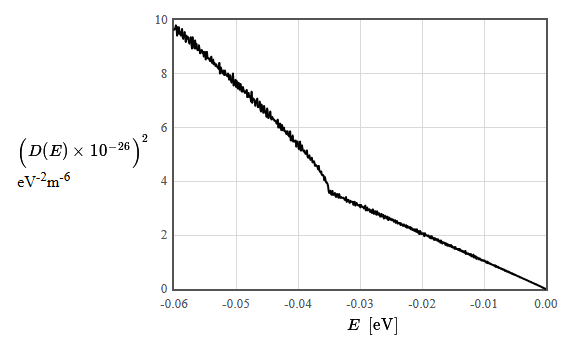### Mechanical properties

The stress-strain relation for silicon is described by the tensor equation,

$\begin{equation} \sigma_{ij}=c_{ijkl}\epsilon_{kl}, \end{equation}$

where the elements of the compliance tensor are,

$c_{11}=165.7$ GPa $=c_{xxxx}=c_{yyyy}=c_{zzzz}$,
$c_{12}=63.9$ GPa $=c_{xxyy}=c_{xxzz}=c_{zzyy}=c_{yyxx}=c_{yyzz}=c_{zzxx}$,
$c_{44}=79.6$ GPa $=c_{xyxy}=c_{xzxz}=c_{yzyz}=c_{yxyx}=c_{zxzx}=c_{zyzy}$,
all other components $c_{ijkl}=0$.

For isotropic materials like silicon, the compliance tensor elements are related to Young's modulus $E$ and Poisson's ratio $\nu$ by

$$c_{11} = \frac{E(1-\nu)}{(1+\nu)(1-2\nu)},$$ $$c_{12} = \frac{E\nu}{(1+\nu)(1-2\nu)},$$ $$c_{44} = \frac{E}{2(1+\nu)}.$$

Yield strength: 7 GPa
Fracture strain: 4%
Possion's ratio: 0.27

When an electric field is applied to a silicon crystal, the resulting strain can be expressed as a Taylor series,

$\begin{equation} \epsilon_{ij}=d_{ijk}E_k+Q_{ijkl}E_kE_l+\cdots, \end{equation}$

where $\epsilon_{ij}$ is the strain, $E_k$ is the electric field, $d_{ijk}$ is the reciprocal piezoelectric tensor, and $Q_{ijkl}$ is the electrostriction tensor. Silicon has inversion symmetry in its point group so the reciprocal piezoelectric tensor is zero by symmetry and the leading order term is electrostriction.

### Optical properties

Dielectric function
The relative dielectric function describes the relationship between the electric displacement $\vec{D}$ and the electric field $\vec{E}$, $\vec{D}=\epsilon_r \epsilon_0 \vec{E}= \vec{P}+\epsilon_0 \vec{E}$.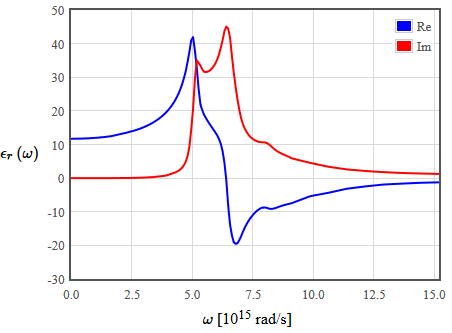There are two conventions for dielectric function. Either it is assumed that the time dependence of $\vec{D}$, $\vec{P}$, and $\vec{E}$ is $\exp (-i\omega t)$ and the plot of the dielectric function looks as it is shown above, or it is assumed that the time dependence of $\vec{D}$, $\vec{P}$, and $\vec{E}$ is $\exp (i\omega t)$ and the imaginary part of the has the opposite sign as in the plot above. Here we will assume a time dependence of $\exp (-i\omega t)$. The data show here was digitized from D. E. Aspnes and A. A. Studna, Phys. Rev. B 27, 985-1009 (1983).

Electric susceptibility
The electric susceptibility $\chi_E$ describes the relationship between the polarization $\vec{P}$ and the electric field $\vec{E}$, $\vec{P} = \epsilon_0\chi_E\vec{E}$.

$\begin{equation} \chi_E= \epsilon_r -1 \end{equation}$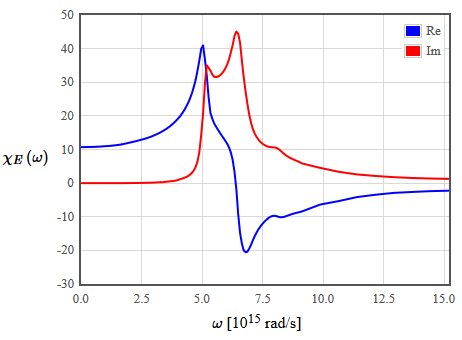Complex conductivity
Assuming that the electric field has a harmonic dependence, $E=E_0 \exp(-i\omega t)$, then the polarization will also have a harmonic dependence, $P = \epsilon_0\chi_E E_0 \exp(-i\omega t)$. The polarization is the charge density $n$ times the charge $q$ times the displacement $x$, $P = nqx = \epsilon_0\chi_E E_0 \exp(-i\omega t)$. Differentiating this with repect to time yields a relationship between the current density $j$ and the electric field, $j = nq\frac{dx}{dt} = -i\omega \epsilon_0\chi_E E$. Since the current density is related to the electric field by the conductivity, $j=\sigma E$, the conductivity is,

$\begin{equation} \sigma\left(\omega \right)=-i\omega \epsilon_0 \chi_E(\omega). \end{equation}$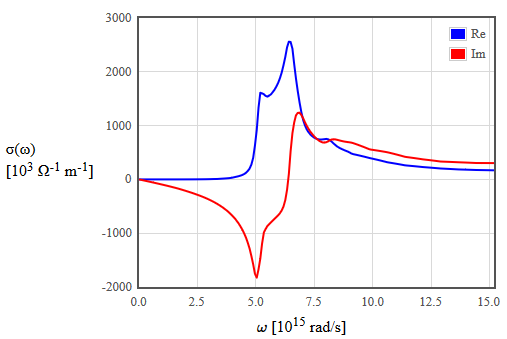The dielectric function, the electric susceptibility, and the complex conductivity are three different ways to present the same information. Usually for frequencies below about 1THz, we use the complex conductivity and for higher frequencies we use the dielectric function or the electric susceptibility.

The index of refraction $n$ and the extinction coefficient $K$
For nonferromagnetic materials where the relative permeability is approximately 1 $(\mu_r\approx 1)$, the real and imaginary parts of the square root of the dielectric function are the index of refraction and the extinction coefficient.

$\begin{equation} \sqrt{\epsilon_r}= n+iK \end{equation}$

When waves travel from vacuum into some material, the frequency remains constant. A plane wave moving to the right in vaccuum has the form $\exp\left(i\left(\omega x/c -\omega t\right)\right)$ where $c$ is the speed of light in vacuum. When this wave enters some material, $c \rightarrow c/ \left(n+iK\right)$. The speed of the electromagnetic waves is smaller than the speed of light in vacuum by a factor of $n$. The extinction coeffcient describes the exponential decay of the amplitude of the electromagnetic waves. For waves propagating in the $x$-direction, the amplitude decays like $\exp\left(-\omega Kx/c \right)$.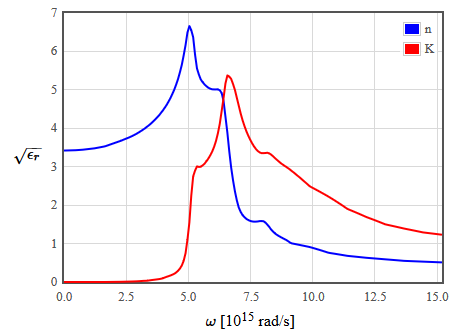Absorption coefficient $\alpha$
The absorption coefficient describes how the intensity of the light decays. Since the intensity is proportional to the amplitude of the waves squared, the exponential decay of the intensity is $I= I_0\exp\left(-\alpha x\right)$ where,

$\begin{equation} \alpha =\frac{2\omega K}{c} \end{equation}$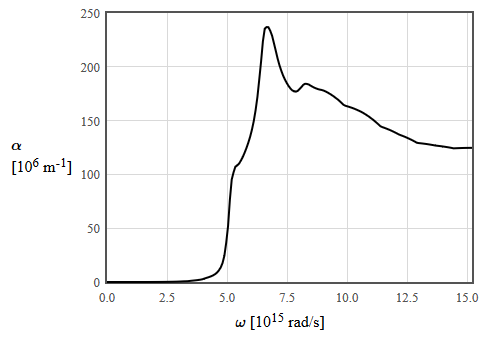Reflection
The reflection coefficient of light striking the crystal normal to the surface from vacuum ($\epsilon_r=1$) is,

$\begin{equation} R=\frac{\left(n-1\right)^2+K^2}{\left(n+1\right)^2+K^2} \end{equation}$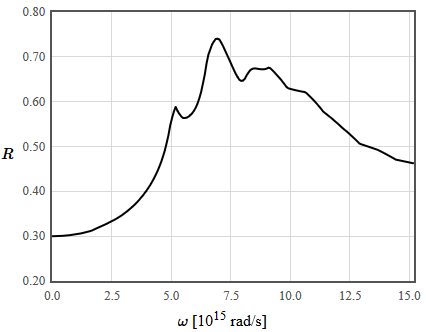[Askerov] B. M. Askerov, Electron Transport Phenomena in Semiconductors, World Scientific (1994).